# Introduction to ggmuller

Ggmuller is an attempt to set an easy-to-use standard for representing evolutionary dynamics as stacked area plots that combine information about frequency dynamics and phylogeny. The horizontal axis is time, and shapes of different colours represent genotypes (or other subtypes), with height corresponding to genotype frequency (or relative abundance) at the corresponding time point. Descendant genotypes are shown emerging from inside their parents.

Such diagrams are sometimes termed Muller plots in honour of Hermann Joseph Muller, who used them in 1932 to illustrate an evolutionary advantage of sex. More recently, they can be seen in experimental evolution papers by Richard Lenski, Jeffrey Barrick and others, and in many cancer evolution reviews.

## How does it work?

The core function is get_Muller_df, which constructs a specially ordered data frame from which we can draw a stacked area plot. get_Muller_df takes two data frames as input: first an adjacency matrix that defines a phylogeny (with columns named “Identity” and “Parent”), and second the populations of each genotype over time (with columns named “Identity”, “Population”, and either “Generation” or “Time”). The genotype names in the “Identity” and “Parent” columns can be any words or numbers. You can use a phylo object instead of an adjacency matrix, provided the genotype names in the population data are integers that correspond to the node numbers in the phylo object.

get_Muller_df records a path through the phylogenetic tree. Each genotype appears exactly twice in the path: once before and once after all of the genotype’s descendants. The function then associates each instance of each genotype with half of the genotype’s frequency over time.

To draw plots, ggmuller exploits Hadley Wickham’s ggplot package (which accounts for the first part of its name). Because the two instances of each genotypes are coloured identically, the resulting plot shows each genotype emerging from the centre of its parent (following Jeffrey Barrick’s example, multiple descendants are stacked from top to bottom in chronological order of appearance).

## Basic usage: frequencies

If your adjacency matrix and your population data frame are properly formatted, you can use ggmuller to visualize your results with just two lines of R code:

library(ggmuller)
Muller_df <- get_Muller_df(example_edges, example_pop_df)
Muller_plot(Muller_df)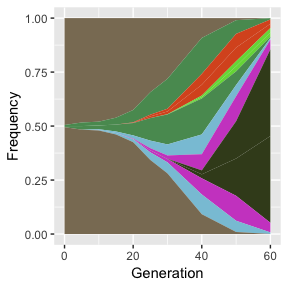Simply replace example_edges and example_pop_df with the names of the data frames that contain your adjacency matrix and population data, respectively.

## Basic usage: population sizes

Whereas Muller_plot shows frequencies, the sister function Muller_pop_plot shows changes in population sizes. Plots of this type are commonly used to show how evolutionary dynamics respond to environmental change, such as in a tumour during chemotherapy. Using the same example data as before:

Muller_df <- get_Muller_df(example_edges, example_pop_df)
Muller_pop_plot(Muller_df)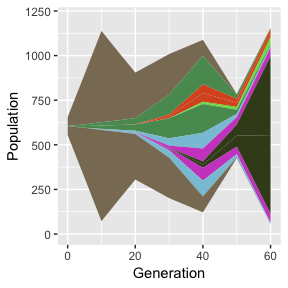That’s all you need for basic usage. The rest of this tutorial introduces some additional features and options.

## A more detailed example

Most of the code that follows is to create an appropriate data set. First we make an adjacency matrix that defines the phylogeny. In this example, the phylogeny is branched such that clone_A begets clone_B and clone_C, then clone_B begets clone_D and clone_E, etc.

edges3 <- data.frame(Parent = paste0("clone_",
LETTERS[c(rep(1:3, each = 2), 2, 5)]),
Identity = paste0("clone_", LETTERS[2:9]))

Next we construct a data frame of genotype populations over time. Here the population data are generated using diverse fitness values:

# a function for generating exponential growth curves:
pop_seq <- function(gens, lambda, start_gen) c(rep(0, start_gen),
exp(lambda * gens[0:(length(gens) - start_gen)]))
lambda <- 0.1 # baseline fitness
gens <- 0:150 # time points
fitnesses <- c(1, 2, 2.2, 2.5, 3, 3.2, 3.5, 3.5, 3.8) # relative fitnesses of genotypes
pop3 <- data.frame(Generation = rep(gens, 9),
Identity = paste0("clone_", LETTERS[rep(1:9, each = length(gens))]),
Population = c(1E2 * pop_seq(gens, fitnesses*lambda, 0),
pop_seq(gens, fitnesses*lambda, 0),
pop_seq(gens, fitnesses*lambda, 10),
pop_seq(gens, fitnesses*lambda, 20),
pop_seq(gens, fitnesses*lambda, 30),
pop_seq(gens, fitnesses*lambda, 40),
pop_seq(gens, fitnesses*lambda, 50),
pop_seq(gens, fitnesses*lambda, 50),
pop_seq(gens, fitnesses*lambda, 60)),
Fitness = rep(fitnesses, each = length(gens)))

Of course there are more concise ways to create this data frame, but the long-winded version makes the data more visible in the code. Note the inclusion of an optional “Fitness” column containing the fitness values. We combine the data using get_Muller_df as before:

Muller_df3 <- get_Muller_df(edges3, pop3)

We create two versions of the plot. The first version is coloured by genotype identity (the default) and has customised axis labels and a legend:

Muller_plot(Muller_df3, add_legend = TRUE, xlab = "Time", ylab = "Proportion")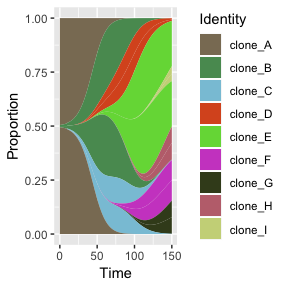The second version is coloured by fitness, making use of that extra column:

Muller_plot(Muller_df3, colour_by = "Fitness", add_legend = TRUE)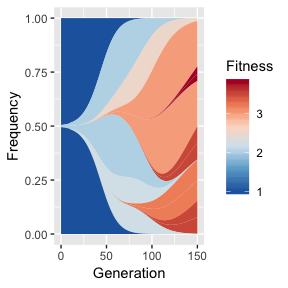Since simulations can result in very large sets of genotypes, many of which never become abundant, get_Muller_df has a cutoff option to exclude rarities:

Muller_df3_censored <- get_Muller_df(edges3, pop3, cutoff = 0.2)
Muller_plot(Muller_df3_censored, add_legend = TRUE)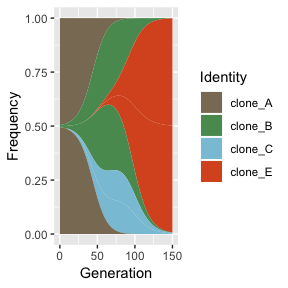## Further customisation with ggplot

Muller_plot is basically a wrapper for ggplot. Although Muller_plot provides some plotting options (palette, axis labels, and whether to include a legend), you may prefer to call ggplot directly to enable further customisation. For example:

library(ggplot2)
my_palette <- c("grey", "red", "magenta", "orange", "yellow", "blue", "darkcyan")
ggplot(Muller_df, aes_string(x = "Generation", y = "Frequency", group = "Group_id", fill = "Identity", colour = "Identity")) +
geom_area() +
theme(legend.position = "right") +
guides(linetype = FALSE, color = FALSE) +
scale_y_continuous(labels = 25 * (0:4), name = "Percentage") +
scale_fill_manual(name = "Identity", values = my_palette) +
scale_color_manual(values = my_palette)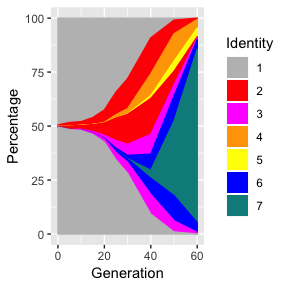You can also use ggplot to produce population plots, but only after using the add_empty_pop function to modify the input data.

Muller_df_pop <- add_empty_pop(Muller_df)
id_list <- sort(unique(Muller_df_pop$Identity)) # list of legend entries, omitting NA ggplot(Muller_df_pop, aes_string(x = "Generation", y = "Population", group = "Group_id", fill = "Identity", colour = "Identity")) + geom_area() + theme(legend.position = "right") + guides(linetype = FALSE, color = FALSE) + scale_fill_manual(name = "Identity", values = my_palette, breaks = id_list) + scale_color_manual(values = my_palette) + theme_classic()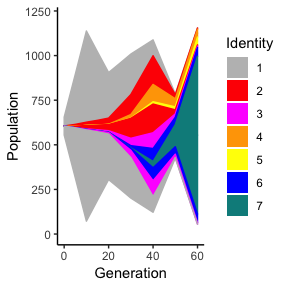## Converting between adjacency matrix and phylo representations We can visualize the tree from our previous example using Emmanuel Paradis’ ape package, but first we need to use the ggmuller function adj_matrix_to_tree to convert our adjacency matrix (with arbitrary node names) to a phylo object that ape can understand. Phylo is the standard way of representing trees in R, and it requires nodes to be numbered in a particular order. tree <- adj_matrix_to_tree(edges3) After optionally labeling the nodes, we use ape’s plot.phylo function to draw the tree: library(ape) tree$tip.label <- 1:length(tree$tip.label) # optional tree$node.label <- (length(tree\$tip.label) + 1):10 # optional
plot(tree, show.node.label = TRUE, show.tip.label = TRUE, tip.color = "red")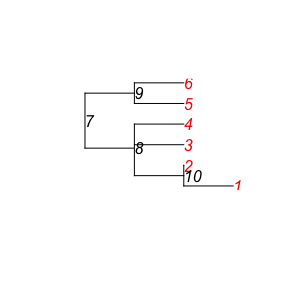If you already have a tree in phylo format, you can use that instead of an adjacency matrix as input to get_Muller_df, as long as the genotype names in the population data are integers that correspond to the node numbers in the phylo object.